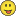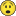# Trying to revise the placement of numbers and letters in a field

1363
12
08-10-2016 01:29 PMNew Contributor

I am new at Python. I have a dbf table with a text field.  Some of the records contain one or two digit numbers at the beginning followed by a string various capital letters which may then be followed by one or two digit numbers and another string of capital letters. Example: 12NESW3SESE. This is just one example of many combinations of numbers and letters. I need to change the order of the records so that the initial one or two digit number follows, rather than precedes, the letters until it gets to the second instance of a one or two digit number. Likewise, the second instance of one or two digit numbers needs to be moved to the end of the second sequence of capital letters. Also, in all cases a semicolon needs to precede the one or two digit number and a comma after the first set of one or two digit numbers and before the second set of capital letters. Hence, the example should be revised to NESW;12,SESE;3

Tags (4)
12 RepliesbyMVP Esteemed Contributor

If they were all the a fixed format in that order, you could keep it simple...but there will be others that have many more creative ways to do this if not fixed.

```aa = "12NESW3SESE"
bb = ("{0};{1};{2};{3}".format(aa[2:6], aa[0:2], aa[7:11], aa[6:7]))
print bb```

Check out The ...py... links   for a link to many others python topics.byMVP Esteemed Contributor

Nice Rebecca... python's mini-formatting language at its best!

The only thing I might add to Rebecca's solution is to sort and select the conditions that meet your requirements.

2 digit number  4 text

1 digit number 4 text   then rearrange

if you happen to get

2 digit number 4 text

2 digit number 4 test   like 12NESW15SESE

then you only have to make a slight change to her formula aa[2:6]], aa[0:2], aa[8:12], aa[6:8]

to account for it.  I suspect you only with have two or at most 3 differing conditions so some big wonking script to do it in one go would be nice, but sometimes a quick select, edit, apply keeps the mind sharpbyMVP Esteemed Contributor

Thanks for the catch...New Contributor

Thanks Rebecca, This is very helpful. Unfortunately, it is not a fixed format. The second record might be something like SW6SWNENENE or even records with no capital letter such as 1. The target format for these two examples would be SW,SWNENENE;6 and simply ;1 , BobbyMVP Honored Contributor

I'm sure this could use some refinement, but I think is approximately what you're after:

```>>> aa = "12NESW3SESE"
... prev_type = aa.isdigit()
... list_1 = []
... cur_word = ''
... for char in aa:
...    if char.isdigit() == prev_type:
...        cur_word += char
...    else:
...        list_1.append(cur_word)
...        cur_word = char
...    prev_type = char.isdigit()
... list_1.append(cur_word)
... print list_1
... list_2 = []
... for i in range(0,len(list_1),2):
...    list_2.append(list_1[i+1] + ';' + list_1)
... print list_2
... final_list = ','.join(list_2)
... print final_list
...
['12', 'NESW', '3', 'SESE']
['NESW;12', 'SESE;3']
NESW;12,SESE;3```byMVP Esteemed Contributor

Darren,   out_list should be list_1 in the code above

```aa = "12NESW3SESE"
prev_type = aa.isdigit()
list_1 = []
cur_word = ''
for char in aa:
if char.isdigit() == prev_type:
cur_word += char
else:
list_1.append(cur_word)
cur_word = char
prev_type = char.isdigit()
list_1.append(cur_word)
print list_1
list_2 = []
for i in range(0,len(list_1),2):
list_2.append(list_1[i+1] + ';' + list_1)
print list_2
final_list = ','.join(list_2)
print final_list```

kept eating my reply...looks like it worked this time.  guess that's what I get for trying to delete one of my other replies!!byMVP Honored Contributor

Oops. thanks. So much for editing for clarity.New Contributor

Thanks Darren, Looks very much like the code I might need. However, as I am still in a steep learning curve, I will have to digest and understand along with other comments to put this to work land records. BobbyMVP Esteemed Contributor

field calculator script... oh well

``````import numpy as np
def shuffle(aa):
""" useage   shuffle(!YourField!) """
a = np.array(list(aa))
b = [int(i.isalpha()) for i in a[:-1]]
c = [int(i.isalpha()) for i in a[1:]]
d = np.sum(np.array(list(zip(b,c))), axis=1)
e = (np.where(d == 1)) + 1
e = e.tolist()
spl = np.split(a,e)
out = []
for s in spl:
out.append("".join([i for i in s]))
out = "{1} {0} {3} {2}".format(*out)
return out
‍‍‍‍‍‍‍‍‍‍‍‍‍‍‍``````

Done verbosely so people can understand

line 04  convert string to a list, then an array

line 05, 06  and 07

do a check for numbers offset by 1 in the sequence  True and False from isalpha is converted to an integer so they can be

added together after forming an n*2 array and summing by row.  This will yield 0,1,2

line 09   all we really care about is where things change, which is where d is 1, but we have to add 1 to the whole sequence

for slicing  (long story, just trust me)

line 10   do the split

lines 12-13  a little list comprehension stuff because they are fast and the data size (aa) is know to be small

line 14    Do the format Rebecca did on the output which you can automagically parse into bits with the *

line 15    send it back

internals

input a   ['1' '2' '3' 'N' 'E' 'S' 'W' '3' '3' 'S' 'E' 'S' 'E']

check b   [0, 0, 0, 1, 1, 1, 1, 0, 0, 1, 1, 1]

check c   [0, 0, 1, 1, 1, 1, 0, 0, 1, 1, 1, 1]

sums  d   [0 0 1 2 2 2 1 0 1 2 2 2]

where e   [3, 7, 9]

split spl NESW 123 SESE 33

sample result

input 123NESW33SESE

output NESW 123 SESE 33

if you don't like the spaces, remove them in line 14 between the {1} {0} etc or you can put commas in whatever.

into a text field

python parser

show code block

copy the above code into the code block

useage in expression box of the field calculator:     shuffle(!YourField!)

You can copy this after line 15 if you just want to test in a script first... I did 2 tests, you should do more

``````#----------------------
if __name__ == "__main__":
"""Main section...  """
aa = "12NESW3SESE"
aa = "123NESW33SESE"
out = shuffle(aa)
print("input {}\noutput {}".format(aa, out))‍‍‍‍‍‍‍``````